## Stabilized solution of the multidimensional advection-diffusion-absorption equation using linear finite elements

E. Oñate, J. Miquel, F. Zárate

Centre Internacional de Metodes Numerics a l'Enginyeria - CIMNE, Barcelona, Spain
Universitat Politècnica de Catalunya (UPC), Barcelona, Spain

## Abstract

A stabilized finite element method (FEM) for the multidimensional steady state advection-diffusion-absorption equation is presented. The stabilized formulation is based on the modified governing differential equations derived via the Finite Calculus (FIC) method. For 1D problems the stabilization terms act as a nonlinear additional diffusion governed by a single stabilization parameter. It is shown that for multidimensional problems an orthotropic stabilizing diffusion must be added along the principal directions of  curvature of the solution. A simple iterative algorithm yielding a stable and accurate solution for all the range of physical parameters and boundary conditions is described. Numerical results for 1D and 2D problems with sharp gradients are presented showing the effectiveness and accuracy of the new stabilized formulation.

## 1 INTRODUCTION

Considerable effort has been spent in recent years to derive finite element methods (FEM) 1 for the solution of the advection-diffusion-reaction equation. In this work we will focus on the so called exponential regime originated by large absorptive (dissipative) reaction terms. Here the solutions are of the form of real exponential functions. Numerical schemes find difficulties to approximating the sharp gradients appearing in the neighborhood of boundary and internal layers due to high Peclet and/or Damköhler numbers. Non physical oscilaltory solution are found with the standard Galerkin FEM unless some stabilization procedure is used.

Stabilized methods to tackle this problem have been based on streamline-upwind/Petrov-Galerkin (SUPG) 2, Galerkin/least-squares 5, Subgrid Scale (SGS) 5 and Residual Free Bubbles 14 finite element methods. While a single stabilization parameter suffices to yield stabilized (and even nodally exact results) for the one-dimensional (1D) advection-diffusion and the diffusion-reaction equations (Vol. 3 in 1 and 8), this is not the case for the diffusion-advection-reaction equation. Here, in general, two stabilization parameters are needed in order to ensure a stabilized solution for all range of physical parameters and boundary conditions 4. As reported in 12 the SUPG, GLS and SGS methods with a single stabilization parameter fail to obtain a stabilized solution for some specific boundary conditions in the exponential regime with negative (absorption) terms when there is a negative streamwise gradient of the solution.

Oñate et al.  have recently presented a stabilized FEM for the advection-diffusion absorption equation based on the use of a single stabilization parameter which has the form of a diffusion term. In  the formulation is detailed for 1D problems and only a brief introduction to the multidimensional case is given. This paper extends the ideas presented in  and provides evidence of the effectiveness and accuracy of the new formulation to deal with multidimensional advection-diffusion-absorption problems with sharp gradients.

The stabilized formulation is based on the standard Galerkin FEM solution of the modified governing differential equations derived via the Finite Calculus (FIC) method [19–20]. The FIC equations are obtained by expressing the balance of fluxes in a domain of finite size. This introduces additional stabilizing terms in the differential equations of the infinitessimal theory which are a function of the balance domain dimensions. Although the FIC–FEM formulation here presented is general, we will restrict its application in this work to linear finite element approximations only.

The Galerkin FIC-FEM formulation described here introduces naturally an additional nonlinear dissipation term into the discretized equations which is governed by a single stabilization parameter. In the absence of the absorption term the formulation simplifies to the standard Petrov-Galerkin approach for the advection-diffusion problem For the diffusion-absorption case the diffusion-type stabilization term is identical to that recently obtained by Felippa and Oñate using a variational FIC approach 15. The general nonlinear form of the stabilization parameter is a function of the signs of the solution and its first and second derivatives. This introduces a non-linearity in the solution scheme and a simple iterative algorithm is described. A simpler constant expression of the stabilization parameter is also presented.

Details of the 1D formulation and its extension to deal with multidimensional problems are given. For the multidimensional case Oñate et al. 27 have recently shown that a general form of the stabilization parameters can be found by writting the FIC equations along the principal curvature directions of the solution. The resulting FIC-FEM formulation is equivalent in this case (for linear elements) to adding a stabilizing diffusion matrix to the standard infinitessimal equation. The stabilizing diffusion matrix depends on the signs of the solution and its derivatives and on the velocities along the principal curvature directions of the solution. This introduces a nonlinearity in the solution process. We present a simple iterative scheme based in assuming that the main principal curvature direction at each point is coincident with the gradient vector direction. In the last part of the paper we present a collection of 1D and 2D examples showing the effectiveness and accuracy of the new FIC-FEM formulation for different values of the advective and absorptive terms.

## 2 FIC FORMULATION OF THE 1D STATIONARY ADVECTION-DIFFUSION-ABSORPTION EQUATION

The governing equation for the 1D stationary advection-diffusion-absorption problem can be written in the FIC formulation as

 $r-{\underline {{h \over 2}{dr \over dx}}}{h \over 2}{dr \over dx}=0\quad {\hbox{in }}x\in (0,L)$
(1)
 $-u\phi +k{d\phi \over dx}+q^{p}-{\underline {{h \over 2}r}}{h \over 2}r=0\quad {\hbox{on }}\Gamma _{q}$
(2)
 $\phi -\phi ^{p}=0\quad {\hbox{on }}\Gamma _{\phi }$
(3)

where

 $r=:-u{d\phi \over dx}+{d \over dx}\left(k{d\phi \over dx}\right)-s\phi +Q$
(4)

In above equations ${\textstyle \phi }$ is the state variable, ${\textstyle x\in [0,L]}$ is the problem domain, ${\textstyle L}$ is the domain length, ${\textstyle u}$ is the velocity field, ${\textstyle k\geq 0}$ is the diffusion, ${\textstyle s\geq 0}$ is the absorption, dissipation or destruction source parameter, ${\textstyle Q}$ is a constant source term, ${\textstyle q^{p}}$ and ${\textstyle \phi ^{p}}$ are the prescribed values of the total flux and the unknown function at the Neumann and Dirichlet boundaries ${\textstyle \Gamma _{q}}$ and ${\textstyle \Gamma _{\phi }}$, respectively and ${\textstyle h}$ is a characteristic length which plays the role of a stabilization parameter. In the 1D problem ${\textstyle \Gamma _{\phi }}$ and ${\textstyle \Gamma _{q}}$ consist of four combinations at the end points of the problem domain.

Eqs.(1) and (2) are obtained by expressing the balance of fluxes in an arbitrary 1D domain of finite size within the problem domain and at the Neumann boundary, respectively. The variations of the transported variables within the balance domain are approximated by Taylor series expansions retaining one order higher terms than in the infinitessimal theory [19,20]. The underlined stabilizing terms in Eqs.(1) and (2) emanate from these higher order expansions. Note that as the characteristic length parameter ${\textstyle h}$ tends to zero the FIC differential equations gradually recover the standard infinitessimal form.

Successful applications of the FIC method to a variety of problems in computational mechanics can be found in [19–30,37].

## 3 FINITE ELEMENT FORMULATION

We will construct a standard finite element discretization ${\textstyle \left\{l^{e}\right\}}$ of the 1D analysis domain length ${\textstyle L}$ with index ${\textstyle e}$ ranging from 1 to the number of elements ${\textstyle N}$ 1. The state variable ${\textstyle \phi }$ is approximated by ${\textstyle {\bar {\phi }}}$ over the analysis domain. The approximated variable ${\textstyle {\bar {\phi }}}$ is interpolated within each element with ${\textstyle n}$ nodes in the standard manner, i.e.

 $\phi \simeq {\bar {\phi }}\quad {\hbox{for}}\quad x\in [0,L]$
(5a)

with

 ${\bar {\phi }}=\sum \limits _{i=1}^{n}N_{i}\phi _{i}$
(5b)

where ${\textstyle N_{i}}$ are the element shape functions and ${\textstyle \phi _{i}}$ are nodal values of the approximate function ${\textstyle {\bar {\phi }}}$. Substituting Eq.(5a) into Eqs.(1) and (2) gives

 ${\bar {r}}-{h \over 2}{d{\bar {r}} \over dx}=r_{\Omega }\quad {\hbox{in }}x\in (0,L)$
 $-u{\bar {\phi }}+k{d{\bar {\phi }} \over dx}+q^{p}-{h \over 2}{\bar {r}}=r_{q}\quad {\hbox{on }}\Gamma _{q}$
(6)
 ${\bar {\phi }}-\phi ^{p}=r_{\phi }\quad {\hbox{on }}\Gamma _{\phi }$
(7)

where ${\textstyle {\bar {r}}=r({\bar {\phi }})}$ and ${\textstyle r_{\Omega },r_{q}}$ and ${\textstyle r_{\phi }}$ are the residuals of the approximate solution in the problem domain and on the Neumann and Dirichlet boundaries ${\textstyle \Gamma _{q}}$ and ${\textstyle \Gamma _{\phi }}$, respectively.

The weighted residual form of Eqs.(6)–(8) is written as

 $\int _{L}W_{i}\left({\bar {r}}-{h \over 2}{d{\bar {r}} \over dx}\right)dx+\left[{\hat {W}}_{i}\left(-u{\bar {\phi }}+k{d{\bar {\phi }} \over dx}+q_{p}-{h \over 2}{\bar {r}}\right)\right]_{\Gamma _{q}}=0$
(8)

where ${\textstyle W_{i}(x)}$ and ${\textstyle {\hat {W}}_{i}}$ are test functions satisfying ${\textstyle W_{i}={\hat {W}}_{i}=0}$ on ${\textstyle \Gamma _{\phi }}$.

Assuming smooth enough solutions and integrating by parts the term involving ${\textstyle h}$ in the first integral gives for ${\textstyle {\hat {W}}_{i}=-W_{i}}$

 $\int _{L}W_{i}{\bar {r}}dx-\left[W_{i}\left(-u{\bar {\phi }}+k{d{\bar {\phi }} \over dx}+q^{p}\right)\right]_{\Gamma _{q}}+\sum \limits _{e}\int _{l^{e}}{h \over 2}{dW_{i} \over dx}{\bar {r}}dx=0$
(9)

The third term in Eq.(10) is computed as the sum of the integrals over the element interiors, therefore allowing for the space derivatives of ${\textstyle {\bar {r}}}$ to be discontinuous. Also in Eq.(10) ${\textstyle h}$ has been assumed to be constant within each element, (i.e. ${\textstyle \displaystyle {dh \over dx}=0}$ within ${\textstyle l^{e}}$).

The weak form is obtained by integrating by parts the advective and diffusive terms within ${\textstyle {\bar {r}}}$ in the first integral of Eq.(10). This gives

 $\int _{L}\left[u{dW_{i} \over dx}{\bar {\phi }}-{dW_{i} \over dx}k{d{\bar {\phi }} \over dx}-W_{i}s{\bar {\phi }}+W_{i}Q\right]dx-[W_{i}q^{p}]_{\Gamma _{q}}-\sum \limits _{e}\int _{l^{e}}\left(\beta k{dW_{i} \over dx}{d{\bar {\phi }} \over dx}-{h \over 2}{dW_{i} \over dx}Q\right)dx=0$
(10)

with

 $\beta =\left[{s{\bar {\phi }} \over 2k{\bar {\phi }}'}+{u \over 2k}-{{\bar {\phi }}'' \over 2{\bar {\phi }}'}\right]h$
(11)

where a prime denotes the derivative with respect to the space coordinate.

Wee see clearly that the last term of Eq.(11) introduces within each element an additional diffusion of value ${\textstyle \beta k}$.

Substituting expression (5b) into (11) and choosing a Galerkin method with ${\textstyle W_{i}=N_{i}}$ within each element gives the discrete system of FE equations written in the standard matrix form as

 ${K}{\bar {\boldsymbol {\phi }}}={f}$
(12)

where ${\textstyle {\bar {\boldsymbol {\phi }}}}$ is the vector of nodal unknowns and the element contributions to matrix K and vector ${\textstyle f}$ are

 $K_{ij}^{e}\!\!$ $=$ $\!\!\int _{l^{e}}\left(-u{dN_{i} \over dx}N_{j}+k(1+\beta ){dN_{i} \over dx}{dN_{j} \over dx}+sN_{i}N_{j}\right)dx$ (13) $f_{i}^{e}\!\!$ $=$ $\!\!\int _{l^{e}}\left(N_{i}+{h \over 2}{dN_{i} \over dx}\right)Qdx-(N_{i}q^{p})_{\Gamma _{q}}$ (14)

The amount of balancing diffusion in Eq.(14) clearly depends on the (nonlinear) stabilization parameter ${\textstyle \beta }$. The element and critical values of ${\textstyle \beta }$ are deduced in the next section for linear two node elements.

We note that the integral of the term ${\textstyle \displaystyle {h \over 2}\displaystyle {dN_{i} \over dx}Q}$ in Eq.(15) vanishes after asssembly when ${\textstyle h}$ and ${\textstyle Q}$ are uniform over a patch of linear elements.

## 4 COMPUTATION OF THE STABILIZATION PARAMETER FOR LINEAR ELEMENTS

The matrix ${\textstyle {K}^{e}}$ and the vector ${\textstyle {f}^{e}}$ for two node linear elements are (for constant values of ${\textstyle u,k}$, ${\textstyle s}$ and ${\textstyle Q}$)

 ${K}^{e}=-{u \over 2}\left[{\begin{matrix}-1&-1\\1&1\\\end{matrix}}\right]+{k \over l^{e}}(1+\beta ^{e})\left[{\begin{matrix}1&-1\\-1&1\\\end{matrix}}\right]+{sl^{e} \over 6}\left[{\begin{matrix}2&1\\1&2\\\end{matrix}}\right]$
(16a)
 ${f}^{e}={Ql^{e} \over 2}\left\{{\begin{matrix}1-\displaystyle {h^{e} \over 2}\\1+\displaystyle {h^{e} \over 2}\\\end{matrix}}\right\}\qquad +\quad {\hbox{boundary term}}$
(16a)

In Eqs.(16) index ${\textstyle e}$denotes element values.

Assuming ${\textstyle Q=0}$, a typical stencil for elements of equal size ${\textstyle l}$ can be written as

 ${\begin{array}{r}-\gamma ({\bar {\phi }}_{i+1}-{\bar {\phi }}_{i-1})-(1+\beta ){\bar {\phi }}_{i-1}+2(1+\beta ){\bar {\phi }}_{i}-(1+\beta ){\bar {\phi }}_{i+1}+\\+\displaystyle {w \over 6}({\bar {\phi }}_{i-1}+4{\bar {\phi }}_{i}+{\bar {\phi }}_{i+1})=0\end{array}}$
(17)

where for simplicity a constant value of ${\textstyle \beta }$ across the mesh has been assumed. In Eq.(17) ${\textstyle \gamma ={ul \over 2k}}$ and ${\textstyle w={sl^{2} \over 4}}$ are the Peclet number and a velocity independent dimensionless number, respectively.

From Eq.(17) we deduce

 $\beta =\gamma \left({{\bar {\phi }}_{i+1}-{\bar {\phi }}_{i-1} \over {\bar {\phi }}_{i+1}-2{\bar {\phi }}_{i}+{\bar {\phi }}_{i-1}}\right)+{w \over 6}\left({{\bar {\phi }}_{i-1}+4{\bar {\phi }}_{i}+{\bar {\phi }}_{i+1} \over {\bar {\phi }}_{i+1}-2{\bar {\phi }}_{i}+{\bar {\phi }}_{i+1}}\right)-1$
(18)

In the vecinity of a sharp gradient zone we can take

 ${\begin{array}{l}{\bar {\phi }}_{i+1}-{\bar {\phi }}_{i-1}\simeq {\bar {\phi }}_{max}S_{1}\\{\bar {\phi }}_{i+1}-2{\bar {\phi }}_{i}+{\bar {\phi }}_{i-1}={\bar {\phi }}_{max}S_{2}\\{\bar {\phi }}_{i}+4{\bar {\phi }}_{i}+{\bar {\phi }}_{i+1}={\bar {\phi }}_{i+1}S_{0}\end{array}}$
(19)

where ${\textstyle {\bar {\phi }}_{max}}$ is the maximum value of the approximate function ${\textstyle {\bar {\phi }}}$ in the patch of elements adjacent to the sharp gradient zone and

 $S_{0}={\hbox{sign }}({\bar {\phi }}),\quad S_{1}={\hbox{sign }}\left({d{\bar {\phi }} \over dx}\right),\quad S_{2}={\hbox{sign }}\left({d^{2}{\bar {\phi }} \over dx^{2}}\right)$
(20)

where sign ${\textstyle {\bar {(\cdot )}}}$ denotes the sign of the magnitude within the brackets computed at the patch mid point.

Substituting Eq.(19) into (18) leads to the following expression of the stabilization parameter

 $\beta =\left[\left({S_{0} \over S_{2}}\right){w \over 6}+\left({S_{1} \over S_{2}}\right)\gamma -1\right]$
(21)

The element stabilization parameter ${\textstyle \beta ^{e}}$ is now defined as

 ${\begin{array}{l}\beta ^{e}=\beta \quad {\hbox{for }}\beta >0\\\beta ^{e}=0\quad {\hbox{for }}\beta \leq 0\end{array}}$
(22)

where ${\textstyle \beta }$ is given by Eq.(21) and the signs ${\textstyle S_{0}}$, ${\textstyle S_{1}}$ and ${\textstyle S_{2}}$ are computed now at the element mid-point.

It is clear from above that the computation of the stabilization parameter ${\textstyle \beta ^{e}}$ requires the knowledge of the sign of the numerical solution ${\textstyle {\bar {\phi }}}$ and that of the first and second derivatives of ${\textstyle {\bar {\phi }}}$ within each element. This necessarily leads to an iterative scheme. A simple algorithm which provides a stabilized and accurate solution in just two steps is presented below.

### 4.1 Critical stabilization parameter and unstability conditions

The following constant value of ${\textstyle \beta }$ over the mesh ensures a stabilized solution for all ranges of ${\textstyle \gamma }$ and ${\textstyle w}$

 $\displaystyle {\beta \leq \beta _{c}={w \over 6}+\vert \gamma \vert -1}$
(23)

where ${\textstyle \beta _{c}}$ is the critical stabilization parameter. Note that ${\textstyle \beta _{c}}$ corresponds to the maximum value of ${\textstyle \beta }$ in Eq.(21) for ${\textstyle {S_{0} \over S_{2}}={S_{1} \over S_{2}}=1}$. A mathematical proof of Eq.(23) is given in .

Clearly the value of ${\textstyle \beta _{c}}$ of Eq.(23) is meaningful only if ${\textstyle \beta _{c}>0}$ and this can be taken as an indicator of an unstable solution. Conversely, a value of ${\textstyle \beta _{c}\leq 0}$ indicates that no stabilization is needed.

### 4.2 Iterative solution scheme

The following two steps iterative scheme is proposed in order to obtain a stabilized and accurate solution.

step step

Compute a first stabilized solution ${\textstyle {\bar {\boldsymbol {\phi }}}^{1}}$ using the critical value ${\textstyle \beta ^{e}=\beta _{c}}$ given by Eq.(23). This ensures a stabilized, although sometimes slightly overdiffusive, solution.

## ~

step

Compute the signs of the first and second derivatives of ${\textstyle {\bar {\boldsymbol {\phi }}}^{1}}$ within each element. The second derivative field is obtained as follows

 $\left({d^{2}{\bar {\phi }}^{1} \over dx^{2}}\right)^{e}={1 \over l^{e}}\left[\left({d{\hat {\phi }}^{1} \over dx}\right)_{2}^{e}-\left({d{\hat {\phi }}^{1} \over dx}\right)_{1}^{e}\right]$
(24)

where ${\textstyle ({\hat {\cdot }})_{i}^{e}}$ denotes averaged values of the first derivative at node ${\textstyle i}$ of element ${\textstyle e}$. At the boundary nodes the constant value of the derivative of ${\textstyle {\bar {\phi }}}$ within the element is taken in Eq.(24); i.e. ${\textstyle ({\hat {\cdot }})_{i}^{e}=\left({d{\bar {\phi }} \over dx}\right)^{(e)}={{\bar {\phi }}_{2}-{\bar {\phi }}_{1} \over l^{e}}}$.

Compute the enhanced stabilized solution ${\textstyle {\boldsymbol {\phi }}^{2}}$ using the element value of ${\textstyle \beta ^{e}}$ given by Eq.(22).

In all the 1D examples solved the above two steps have sufficed to obtain a converged stabilized and accurate solution . The reason of this is that the signs of the first and second derivative fields typically do not change any further after the second step over the elements adjacent to high gradient zones.

## 5 EXTENSION TO MULTI-DIMENSIONAL PROBLEMS

Consider the general steady-state advection-diffusion-reaction equation written for the zero constant source case (${\textstyle Q=0}$) as

 $r(\phi ):=-{u}^{T}{\boldsymbol {\nabla }}\phi +{\boldsymbol {\nabla }}^{T}{D}{\boldsymbol {\nabla }}\phi -s\phi =0$
(25)

For 2D problems

 ${u}=[u,v]^{T}\quad ,\quad {\boldsymbol {\nabla }}=\left[{\partial \over \partial x},{\partial \over \partial y}\right]^{T}\quad ,\quad {D}=k\left[{\begin{matrix}1&0\\0&1\\\end{matrix}}\right]$
(26)

are respectively the velocity vector, the gradient vector and the diffusivity matrix, respectively. For simplicity we have assumed an isotropic diffusion matrix.

The FIC form of Eq.(25) is written as

 $r-{\underline {{1 \over 2}{h}^{T}{\boldsymbol {\nabla }}r}}{1 \over 2}{h}^{T}{\boldsymbol {\nabla }}r=0$
(27)

where ${\textstyle r}$ is the original infinitessimal differential equation as defined in Eq.(25).

The Dirichlet and boundary conditions of the FIC formulation are

 $\phi -\phi ^{p}=0\quad {\hbox{on}}\quad \Gamma _{\phi }$
(28)

 $-{u}^{T}{n}\phi +{n}^{T}{D}{\boldsymbol {\nabla }}\phi +q^{p}-{\underline {{1 \over 2}{h}^{T}{n}r}}{1 \over 2}{h}^{T}{n}r=0\quad {\hbox{on}}\quad \Gamma _{q}$
(29)

where ${\textstyle n}$ is the normal vector to the boundary where the normal flux is prescribed. As usual index ${\textstyle p}$ denotes the prescribed values.

In Eqs.(27) and (29) ${\textstyle {h}=[h_{x},h_{y}]^{T}}$ is the characteristic vector of the 2D FIC formulation which components play the role of stabilization parameters. The underlined terms in Eqs.(27) and (29) introduce the necessary stability in the numerical solution [19,20,21].

If vector h is taken to be parallel to the velocity u the standard SUPG method is recovered [18–23]. The more general form of h allows to obtain stabilized finite element solutions in the presence of strong gradients of ${\textstyle \phi }$ near the boundaries (boundary layers) and within the analysis domain (internal layers) 27. The FIC formulation therefore reproduces the best features of the so called shock-capturing or transverse-dissipation schemes 2.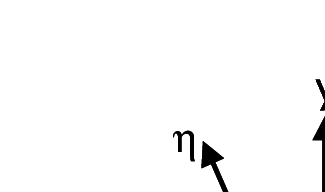Global axes ($x,y$) and principal curvature axes ($\xi ,\eta$)

Let us write down the FIC balance equation in the principal curvature axes of the solution ${\textstyle \xi ,\eta }$ (Figure 1). The FIC balance equation is

 $-u_{\xi }{\partial \phi \over \partial \xi }-u_{\eta }{\partial \phi \over \partial \eta }+k\left({\partial ^{2}\phi \over \partial \xi ^{2}}+{\partial ^{2}\phi \over \partial \eta ^{2}}\right)-s\phi -{h_{\xi } \over 2}{\partial \over \partial \xi }\left[-u_{\xi }{\partial \phi \over \partial \xi }-u_{\eta }{\partial \phi \over \partial \eta }+k\left({\partial ^{2}\phi \over \partial \xi ^{2}}+{\partial ^{2}\phi \over \partial \eta ^{2}}\right)-s\phi \right]$ $-{h_{\eta } \over 2}{\partial \over \partial \eta }\left[-u_{\xi }{\partial \phi \over \partial \xi }-u_{\eta }{\partial \phi \over \partial \eta }+k\left({\partial ^{2}\phi \over \partial \xi ^{2}}+{\partial ^{2}\phi \over \partial \eta ^{2}}\right)-s\phi \right]=0$
(30)

where ${\textstyle u_{\xi },u_{\eta }}$ are the velocities along the principal axes of curvature ${\textstyle \xi }$ and ${\textstyle \eta }$, respectively.

As ${\textstyle \xi }$ and ${\textstyle \eta }$ are the principal curvature axes of the solution then

 ${\partial ^{2}\phi \over \partial \xi \partial \eta }={\partial ^{2}\phi \over \partial \eta \partial \xi }=0$
(31)

Introducing this simplification into Eq.(30) we can rewrite this equation as

 ${\begin{array}{l}-u_{\xi }\displaystyle {\partial \phi \over \partial \xi }-u_{\eta }{\partial \phi \over \partial \eta }+\left(k+{u_{\xi }h_{\xi } \over 2}+{sh_{\xi } \over 2}{\partial \phi \over \partial \xi }\left({\partial ^{2}\phi \over \partial \xi ^{2}}\right)^{-1}\right){\partial ^{2}\phi \over \partial \xi ^{2}}+\\\displaystyle +\left(k+{u_{\eta }h_{\eta } \over 2}+{sh_{\eta } \over 2}{\partial \phi \over \partial \eta }\left({\partial ^{2}\phi \over \partial \eta ^{2}}\right)^{-1}\right){\partial ^{2}\phi \over \partial \eta ^{2}}-s\phi -k\left({h_{\xi } \over 2}{\partial ^{3}\phi \over \partial \xi ^{3}}+{h_{\eta } \over 2}{\partial ^{3}\phi \over \partial \eta ^{3}}\right)=0\end{array}}$
(32a)

or

 $-u_{\xi }{\partial \phi \over \partial \xi }-u_{\eta }{\partial \phi \over \partial \eta }+k(1+\beta _{\xi }){\partial ^{2}\phi \over \partial \xi ^{2}}+k(1+\beta _{\eta }){\partial ^{2}\phi \over \partial \eta ^{2}}-s\phi -k\left({h_{\xi } \over 2}{\partial ^{3}\phi \over \partial \xi ^{3}}+{h_{\eta } \over 2}{\partial ^{3}\phi \over \partial \eta ^{3}}\right)=0$
(32b)

We can see clearly from Eq.(33) that the FIC governing equations introduce orthotropic diffusion parameters of values ${\textstyle {\beta _{\xi }k}}$ and ${\textstyle {\beta _{\eta }k}}$ along the ${\textstyle \xi }$ and ${\textstyle \eta }$ axes, respectively with

 $\beta _{\xi }={u_{\xi }h_{\xi } \over 2k}+{sh_{\xi } \over 2k}{\partial \phi \over \partial \xi }\left({\partial ^{2}\phi \over \partial \xi ^{2}}\right)^{-1},\quad \beta _{\eta }={u_{\xi }h_{\xi } \over 2k}+{sh_{\eta } \over 2k}{\partial \phi \over \partial \eta }\left({\partial ^{2}\phi \over \partial \eta ^{2}}\right)^{-1}$
(33)

Also note that the last term of Eq.(32b) will vanish after discretization for linear elements.

Eq.(32b) can be rewritten in matrix form (neglecting the last term) as

 $-{u}^{\prime T}{\boldsymbol {\nabla }}^{\prime }\phi +{\boldsymbol {\nabla }}^{\prime T}({D}+{\bar {D}}^{\prime }){\boldsymbol {\nabla }}^{\prime }\phi -s\phi =0$

where ${\textstyle {u}^{\prime }=[u_{\xi },u_{\eta }]^{T}}$, ${\textstyle {\boldsymbol {\nabla }}^{\prime }=\left[{\partial \over \partial \xi },{\partial \over \partial \eta }\right]^{T}}$, ${\textstyle D}$ is the “physical” isotropic diffusion matrix and ${\textstyle {\bar {D}}'}$ is the balancing diffusion matrix in the local axes ${\textstyle \xi }$ and ${\textstyle \eta }$. The form of this matrix is

 ${\bar {D}}^{\prime }=\left[{\begin{array}{cc}\beta _{\xi }k&0\\0&\beta _{\eta }k\end{array}}\right]$
(34)

The velocities along the principal curvature axes ${\textstyle u_{\xi }}$ and ${\textstyle u_{\eta }}$ can be obtained by projecting the cartesian velocities into the principal curvature axes ${\textstyle \xi }$ and ${\textstyle \eta }$ as

 ${u}'=\left\{{\begin{array}{c}u_{\xi }\\u_{\eta }\end{array}}\right\}={T}{u}\quad {\hbox{with}}\quad {T}=\left[{\begin{array}{cc}c_{\alpha }&s_{\alpha }\\-s_{\alpha }&c_{\alpha }\end{array}}\right]\quad ,\quad {u}=\left\{{\begin{array}{c}u\\v\end{array}}\right\}$
(35)

where ${\textstyle c_{\alpha }=\cos \alpha }$, ${\textstyle s_{\alpha }=\sin \alpha }$ and ${\textstyle \alpha }$ is the angle which the ${\textstyle \xi }$ axis forms with the ${\textstyle x}$ axis (Figure 1). Note that as the solution is continuous the principal curvature directions ${\textstyle \xi }$ and ${\textstyle \eta }$ are orthogonal.

The values of ${\textstyle \beta _{\xi }}$ and ${\textstyle \beta _{\eta }}$ are computed by considering the solution of two uncoupled 1D problems along the ${\textstyle \xi }$ and ${\textstyle \eta }$ directions. This gives from Eqs.(21) and (22)

 $\beta _{\xi }=\left[\left({S_{0} \over S_{\xi _{2}}}\right){w_{\xi } \over 6}+\left({S_{\xi _{1}} \over S_{\xi _{2}}}\right)\gamma _{\xi }-1\right]\quad ,\quad \gamma _{\xi }={u_{\xi }l_{\xi } \over 2k}\quad ,\quad w_{\xi }={sl_{\xi }^{2} \over k}$
(37a)
 $\beta _{\eta }=\left[\left({S_{0} \over S_{\eta _{2}}}\right){w_{\eta } \over 6}+\left({S_{\eta _{1}} \over S_{\eta _{2}}}\right)\gamma _{\eta }-1\right]\quad ,\quad \gamma _{\eta }={u_{\eta }l_{\eta } \over 2k}\quad ,\quad w_{\eta }={sl_{\eta }^{2} \over k}$
(37b)

where

 ${\begin{array}{l}S_{0}={\hbox{sign }}({\bar {\phi }})\quad ,\quad S_{\xi _{1}}={\hbox{sign }}\left(\displaystyle {\partial {\bar {\phi }} \over \partial \xi }\right)\quad ,\quad S_{\xi _{2}}={\hbox{sign }}\left(\displaystyle {\partial ^{2}{\bar {\phi }} \over \partial \xi ^{2}}\right)\\S_{\eta _{1}}={\hbox{sign }}\left(\displaystyle {\partial {\bar {\phi }} \over \partial \eta }\right)\quad ,\quad S_{\eta _{2}}={\hbox{sign }}\left(\displaystyle {\partial ^{2}{\bar {\phi }} \over \partial \eta ^{2}}\right)\end{array}}$
(38)

and ${\textstyle {\bar {\phi }}}$ is as usual the approximate solution.

The lengths ${\textstyle l_{\xi }}$ and ${\textstyle l_{\eta }}$ are taken as the maximum projection of the velocities ${\textstyle u_{\xi }}$ and ${\textstyle u_{\eta }}$ along the element sides (for triangles) and the element diagonals (for quadrilaterals), i.e.

 $l_{i}=\max({d}_{j}^{T}{u}_{i})\quad ,\quad i=\xi ,\eta$
(39a)

with

 ${\begin{array}{ll}j=1,2,3{\hbox{ (for triangles) and }}\\j=1,2{\hbox{ (for quadrilaterals)}}\end{array}}$
(39b)

In Eq.(39a) ${\textstyle {u}_{\xi }}$ and ${\textstyle {u}_{\eta }}$ contain the global components of the velocity vectors ${\textstyle {\vec {u}}_{\xi }}$ and ${\textstyle {\vec {u}}_{\eta }}$, respectively. For triangles ${\textstyle {d}_{j}}$ are the element side vectors, whereas for quadrilaterals ${\textstyle {d}_{j}}$ are the element diagonal vectors 27.

The next step is to transform Eq.(34) to global axes ${\textstyle x,y}$. The resulting equation is

 $-{u}^{T}{\boldsymbol {\nabla }}\phi +{\boldsymbol {\nabla }}^{T}{D}_{G}{\boldsymbol {\nabla }}\phi -s\phi =0$

where the global diffusion matrix ${\textstyle {D}_{G}}$ is given by

 ${D}_{G}={D}+{\bar {D}}$
(41a)

where the global balancing diffusion matrix ${\textstyle {\bar {D}}}$ is

 ${\bar {D}}={T}^{T}{\bar {D}}^{\prime }{T}$
(41b)

### Remark

Similarly as for the 1D problems, a negative value of the parameters ${\textstyle \beta _{\xi }}$ and ${\textstyle \beta _{\eta }}$ indicates that no stabilization is needed along the directions ${\textstyle \xi }$ and ${\textstyle \eta }$, respectively. A zero value of the corresponding stabilization parameter is chosen in this case.

### Remark

The expressions of ${\textstyle \beta _{\xi }}$ and ${\textstyle \beta _{\eta }}$ in Eq.(37) can be simplified to

 ${\begin{array}{l}\beta _{\xi }\simeq \beta _{\xi _{c}}=\left[\displaystyle {w_{\xi } \over 6}+|\gamma _{\xi }|-1\right]\\\beta _{\eta }\simeq \beta _{\eta _{c}}=\left[\displaystyle {w_{\eta } \over 6}+|\gamma _{\eta }|-1\right]\end{array}}$
(42)

This avoids the computation of the sign of the solution and of its first and second derivatives. The expressions of ${\textstyle \beta _{\xi _{c}}}$ and ${\textstyle \beta _{\eta _{c}}}$ in Eq.(42) are equivalent to that of the 1D critical stabilization parameter ${\textstyle \beta _{c}}$ of Eq.(23). The main difference is that the computation of the local directions ${\textstyle \xi }$ and ${\textstyle \eta }$ is still mandatory in the multidimensional case and, therefore, the nonlinearity of the process can not be avoided here.

### 5.1 Computation of the principal curvature axes for linear elements

Excellent results have been obtained in our work by approximating the main curvature direction ${\vec {\xi }}$ by the direction of the gradient vector ${\textstyle {\boldsymbol {\nabla }}\phi }$.

This simplification allows us to estimate the direction ${\textstyle {\vec {\xi }}}$ in a very economical manner as the gradient vector ${\textstyle {\boldsymbol {\nabla }}{\bar {\phi }}}$ can be directly computed at any point of a linear element. Direction ${\textstyle {\vec {\eta }}}$ is taken orthogonal to that of ${\textstyle {\vec {\xi }}}$ in an anti-clockwise sense.

For linear triangles ${\textstyle {\boldsymbol {\nabla }}{\bar {\phi }}}$ is constant within the element. For four node quadrilaterals ${\textstyle {\boldsymbol {\nabla }}{\bar {\phi }}}$ varies linearly. We have assumed in this case that the direction of ${\textstyle {\vec {\xi }}}$ is constant within the element and equal to the direction of vector ${\textstyle {\boldsymbol {\nabla }}{\bar {\phi }}}$ computed at the element center.

The computation of the signs of the second derivative of ${\textstyle {\bar {\phi }}}$ in Eq.(38) involves the following steps: 1) recovery of the cartesian first derivative field at the nodes using a nodal averaging procedure; 2) computation of the second derivative tensor at the element center and 3) transformation of this tensor to the local axes ${\textstyle \xi }$ and ${\textstyle \eta }$.

We note that in problems where positive values of ${\textstyle {\bar {\phi }}}$ are prescribed at the Dirichlet boundary, the signs of ${\textstyle S_{\xi _{2}}}$, ${\textstyle S_{\eta _{2}}}$ are positive due to the convexity of the numerical solution.

As mentioned above the dependence of the balancing diffusion matrix ${\textstyle {\bar {D}}}$ with the principal curvature directions ${\textstyle {\vec {\xi }}}$ and ${\textstyle {\vec {\eta }}}$ introduces a nonlinearity in the solution process. A simple and effective iterative algorithm is described next.

### 5.2 General iterative scheme

A stabilized numerical solution can be found by the following algorithm.

Step 1 . For elements in the interior of the domain choose ${\textstyle {}^{1}{\boldsymbol {\xi }}={u}}$, i.e. the gradient direction is taken coincident with the velocity direction. If ${\textstyle {u}=0}$ then ${\textstyle {}^{1}{\boldsymbol {\xi }}}$ is taken coincident with the global ${\textstyle {x}}$ axis.

In elements adjacent to a boundary choose ${\textstyle {}^{1}{\boldsymbol {\xi }}={n}}$ where n is the normal to the boundary.

Compute ${\textstyle {}^{1}{\boldsymbol {\eta }},{}^{1}{\bar {D}}'}$, ${\textstyle {}^{1}{\bar {D}}}$ and ${\textstyle {}^{1}{D}_{G}}$ using the expressions of ${\textstyle \beta _{\xi }}$ and ${\textstyle \beta _{\eta }}$ from Eq.(42).

Solve for ${\textstyle {}^{1}{\bar {\boldsymbol {\phi }}}}$.

Verify that the solution is stable. This implies that there are not undershoots or overshoots in the numerical results with respect to the expected physical values. If the numerical solution is unstable, then go to step 2.

Step 2 . For all elements, compute at the element center ${\textstyle {}^{2}{\boldsymbol {\xi }}={\boldsymbol {\nabla }}^{1}{\bar {\phi }}}$. Then compute ${\textstyle {}^{2}{\boldsymbol {\eta }},{}^{2}{\bar {D}}'}$, ${\textstyle {}^{2}{\bar {D}}}$ and ${\textstyle {}^{2}{D}_{G}}$ using the expressions of ${\textstyle \beta _{\xi }}$ and ${\textstyle \beta _{\eta }}$ from Eqs.(37).

Solve for ${\textstyle {}^{2}{\bar {\boldsymbol {\phi }}}}$.

Step 3 . Estimate the convergence of the process. We have chosen the following convergence norm

 $\Vert \phi \Vert ={1 \over N{\bar {\phi }}_{max}}\left[\sum \limits _{j=1}^{n}\left({}^{i}{\bar {\phi }}_{j}-{}^{i-1}{\bar {\phi }}_{j}\right)^{2}\right]^{1/2}\leq \varepsilon$
(43)

where ${\textstyle N}$ is the total number of nodes in the mesh and ${\textstyle \phi _{max}}$ is the maximum prescribed value at the Dirichlet boundary (if ${\textstyle {\bar {\phi }}_{max}=0}$ then ${\textstyle {\bar {\phi }}_{max}=1}$). In above steps the left upper indices denote the iteration number.

In the examples shown in the next section ${\textstyle \varepsilon =10^{-3}}$ has been taken.

If condition (43) is not satisfied, repeat steps 2 and 3 until convergence.

### Remark

For the advective-diffusive problems (i.e. ${\textstyle s=0}$) the expression of the balancing diffusion matrix in the interior elements for step 1 coincides with the standard (linear) SUPG form 27.

### Remark

An alternative solution scheme is to use a time relaxation technique based in the solution of a pseudo transient problem with a forward Euler scheme and a diagonal mass matrix.

## 6 1D NUMERICAL EXAMPLES

The examples presented in this section are solved in a 1D domain discretized with eight two-node linear elements of unit size. The examples are solved with the same Dirichlet conditions ${\textstyle \phi _{1}^{p}=8}$ and ${\textstyle \phi _{9}^{p}=3}$ and two different values of ${\textstyle \gamma }$ and ${\textstyle w}$ (${\textstyle \gamma =1,w=20}$ and ${\textstyle \gamma =10,w=20}$). We note that for both cases the instability condition (${\textstyle \beta _{c}>0}$) is violated and, hence, the Galerkin solution should yield non-physical results.

Figures 2 and 3 show the numerical results obtained with the standard Galerkin method (${\textstyle \beta =0}$) and using the element (${\textstyle \beta ^{e}}$) and critical (${\textstyle \beta _{c}}$) values of the stabilization parameter given by Eqs.(22) and (23), respectively. The exact analytical solution is also shown for comparison.

Table 1 shows the nodal values of the results of the example of Figure 3 for comparison with the 2D solutions presented in the next section.

The following conclusions are drawn from the 1D results.

1. The Galerkin solution (${\textstyle \beta =0}$) is unstable for both problems, as expected.
2. The solution obtained with the critical value ${\textstyle \beta _{c}}$ is always stable, although it yields slightly overdiffusive results in both cases.
3. The results obtained with ${\textstyle \beta ^{e}}$ are less diffusive and more accurate than those obtained with ${\textstyle \beta _{c}}$. The explanation is that the sign of the ratio ${\textstyle {S_{1}/S_{2}}}$ is negative in the region close to the left end point of the domain. This naturally reduces the value of the stabilizing diffusion parameter ${\textstyle \beta }$ in Eq.(21) with respect to that of ${\textstyle \beta _{c}}$ in Eq.(23) where the sign effect is not relevant.
4. The FIC algorithm provides a stabilized solution for Dirichlet problems when there is a negative streamwise gradient of the solution. This is an advantage versus SUPG, GLS and SGS methods using a single stabilization parameter which fail in some cases for these type of problems 12.

Above conclusions have been confirmed in the solution of a wider collection of 1D problems presented in .$\phi _{1}^{p}=8,\phi _{9}^{p}=3,\gamma =1$ and $\omega =20$. FIC results for a mesh of 8 linear elements obtained for $\beta =0$ (Galerkin), $\beta ^{e}$ and $\beta _{c}$. Comparison with the analytical solution.$\phi _{1}^{p}=8,\phi _{9}^{p}=3,\gamma =10$ and $\omega =20$. FIC results for a mesh of 8 linear elements obtained for $\beta =0$ (Galerkin), $\beta ^{e}$ and $\beta _{c}$. Comparison with the analytical solution.

## 7 2D EXAMPLES

The analysis domain in the first two 2D examples presented is a square of size 8 units. The problems are solved first with relatively coarse meshes of ${\textstyle 8\times 8}$ four node bi-linear square elements and ${\textstyle 8\times 8\times 2}$ linear triangles.

The boundary conditions for both examples are ${\textstyle \phi ^{p}=8}$ and ${\textstyle \phi ^{p}=3}$ at the boundaries ${\textstyle x=0}$ and ${\textstyle x=8}$, respectively and zero normal flux at ${\textstyle y=0}$ and ${\textstyle y=8}$. This reproduces the condition of the two 1D examples solved in the previous section. The first example is analized for ${\textstyle {u}=[2,0]^{T}}$, ${\textstyle k=1}$ and ${\textstyle s=20}$ giving ${\textstyle w=20}$, ${\textstyle \gamma _{x}=1}$ and ${\textstyle \gamma _{y}=0}$ which corresponds to the first 1D example (Figure 2). The correct solution for this problem has a boundary layer in the vecinity of the two sides at ${\textstyle x=0}$ and ${\textstyle x=8}$ where ${\textstyle \phi }$ is prescribed (Figure 4). The numerical results obtained with the standard Galerkin solution are oscillatory as expected. The stabilized FIC formulation elliminates the oscillations and yields the correct physical solution. Good results are obtained for both meshes of linear rectangles and triangles (Figures 4 and 5).

Results labelled as FIC-1 and FIC-2 in the figures correspond to those obtained in the first and second iteration of the algorithm presented in Section 5.2, respectively. We note that the FIC-1 results agree precisely with those obtained in the 1D case for ${\textstyle \beta =\beta _{c}}$, whereas the FIC-2 results agree with the more accurate 1D values obtained with the element stabilization parameter ${\textstyle \beta ^{e}}$ (see Figure 2).

The second example is similar to the first one with ${\textstyle {u}=[20,0]^{T}}$, ${\textstyle k=1}$ and ${\textstyle s=20}$ giving ${\textstyle w=20}$, ${\textstyle \gamma _{x}=10}$ and ${\textstyle \gamma _{y}=0}$. These values correspond to the second 1D problem of the previous section (Figure 3). The Galerkin solution is again oscillatory, whereas the FIC results are physically sound (Figures 6 and 7). Once more the FIC-1 and FIC-2 results are in good agreement with the 1D values shown in Figure 3 for ${\textstyle \beta _{c}}$ and ${\textstyle \beta _{e}}$, respectively for both meshes of square and triangular elements. The coincidence of the 1D and 2D results for this problem can be clearly seen in Table 1.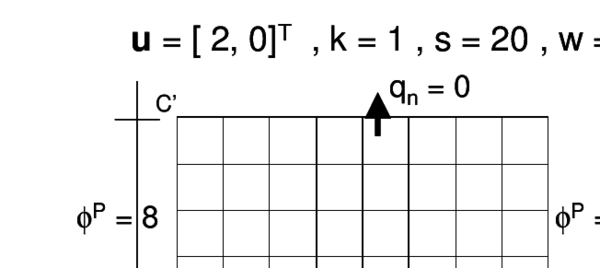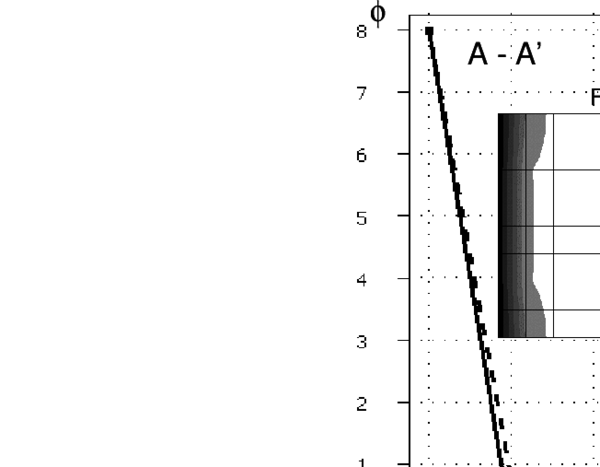2D advection-conduction-absorption problem over a square domain of size equal to 8 units. $\phi ^{p}=8$ at $x=0$, $\phi ^{p}=3$ at $x=8$, $q_{n}=0$ at $y=0$ and $y=8$. ${u}=[2,0]^{T}$, $k=1$, $s=20$, $w=20$, $\gamma _{x}=1$ and $\gamma _{y}=0$. Galerkin and FIC solutions for a mesh of $8\times 8$ four node square elements.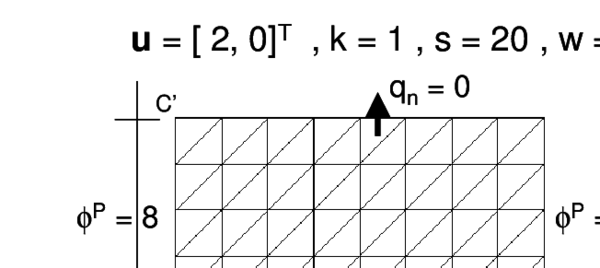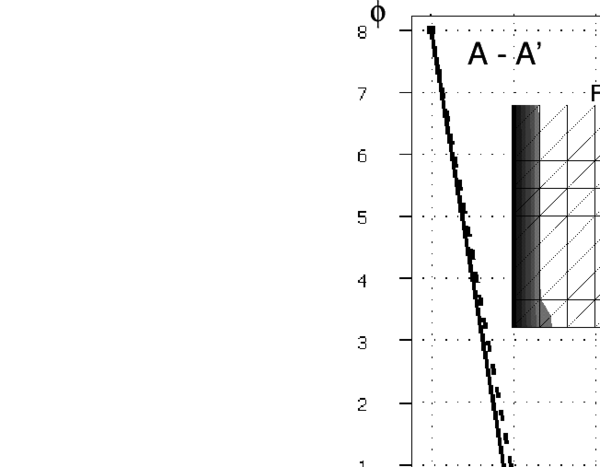Solution of problem of Figure 4 with a mesh of $8\times 8\times 2$ linear triangles.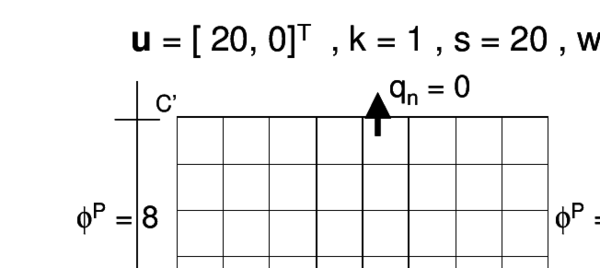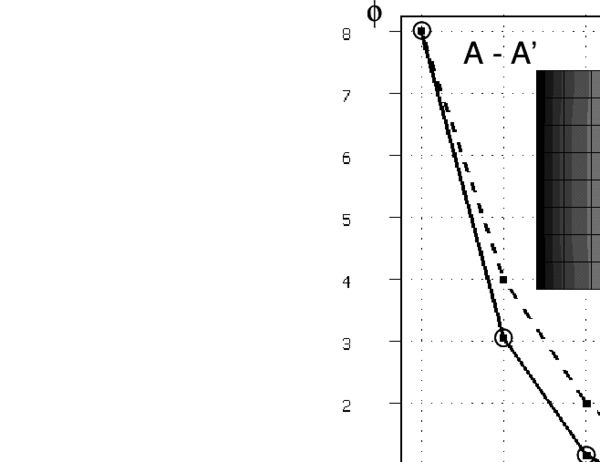2D advection-conduction-absorption problem over a square domain of size equal to 8 units. $\phi ^{p}=8$ at $x=0$, $\phi ^{p}=3$ at $x=8$, $q_{n}=0$ at $y=0$ and $y=8$. ${u}=[20,0]^{T}$, $k=1$, $s=20$, $w=20$, $\gamma _{x}=10$ and $\gamma _{y}=0$. Galerkin and FIC solutions for a mesh of $8\times 8$ four node square elements.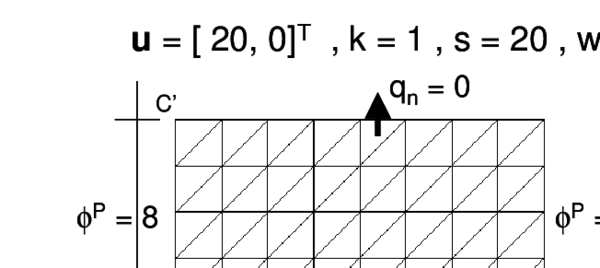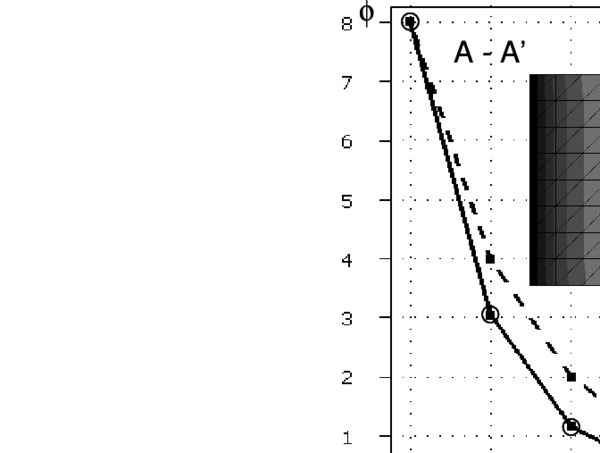Solution of problem of Figure 5 with a mesh of $8\times 8\times 2$ linear triangles.

 1D 2D (nodes along line A-A') Figure 3 4 node quads. (Fig. 6) 3 node triangles (Fig. 7) Node ${\bar {\phi }}(\beta =0$) ${\bar {\phi }}(\beta ^{e}$) ${\bar {\phi }}(\beta _{c}$) $\phi$(exact) FIC-1 FIC-2 FIC-1 FIC-2 1 8,00 8 8 8 8 8 8 8 2 2,94 3,06 4 3,08 3,99 3,057 4,0 3,059 3 1,32 1,17 2 1,19 2,00 1,170 2,0 1,167 4 1,80 0,447 1 0,457 1,00 0,448 1,0 0,452 5 0,599 0,172 0,5 0,176 0,49 0,172 0,499 0,166 6 -0,633 0,0646 0,25 0,0677 0,248 0,0648 0,2501 0,0681 7 1,16 0,0264 0,125 0,0261 0,125 0,0255 0,1250 0,0257 8 -1,83 0,0073 0,0625 0,01 0,0615 0,0101 0,0624 0,0072 9 3 3 3 3 3 3 3 3

Note that, similarly to the 1D case, the FIC-2 results are more accurate (less diffusive) than those obtained in the first iteration (FIC-1). This is due to the more precise evaluation of ${\textstyle \beta _{s}}$ and ${\textstyle \beta _{\eta }}$ in Eqs.(37) accounting for the correct sign of all the terms.

Figures 8–11 show results for the two 2D problems above described solved now with relatively coarse unstructured meshes of linear triangles and quadrilaterals. The effectiveness and accuracy of the FIC iterative scheme is again noticeable in all cases. Note the agreement of the FIC-2 results of Figures 10 and 11 with the exact solution for the equivalent 1D problem of Figure 3.

Figure 12 presents the solution of a similar problem where the values of ${\textstyle \phi }$ are prescribed at the four boundaries. The solution domain has now 10 units and the problem is solved first with a mesh of ${\textstyle 10\times 10}$ four node square elements. Details of the physical parameters are given in Figure 12. Excellent results are again obtained with the FIC scheme. Similar good results are obtained with a structured mesh of linear triangles (Figure 13) as well as with non structured meshes of linear quadrilateral and triangles (Figures 14 and 15).

The effectiveness of the FIC scheme for a diffusive-absorptive problem with Dirichlet boundary conditions is shown in Figure 16. The results shown have been obtained with structured meshes of linear quadrilateral and triangles. Note that the four boundary layers are well captured in the first step of the iterative solution. Similar good results have also been obtained with unstructured meshes not shown here.

The final example is a standard benchmark problem of advection-diffusion where sharp layers appear at both the boundary and the interior of the domain. The problem is the advective-diffusive transport of ${\textstyle \phi }$ in a square domain with non uniform Dirichlet conditions, downwards diagonal velocity and zero source terms (i.e. ${\textstyle Q=0}$ and ${\textstyle s=0}$). Figure 17 displays the SUPG solution and FIC results obtained after two iterations using a structured mesh of ${\textstyle 20\times 20}$ linear four node square elements. It is remarkable that the FIC results capture the sharp gradient zones at the boundaries where ${\textstyle \phi }$ is prescribed to zero and at the interior of the domain and elliminate all the spurious oscillations present in the SUPG method.

Similar good results obtained with the FIC method for a wide range of advective-diffusive problems are presented in 27. Recent applications of the FIC method to incompressible fluid flow problems are reported in .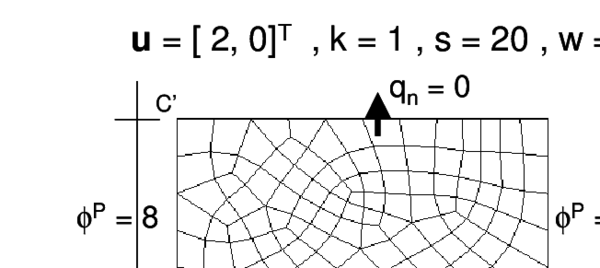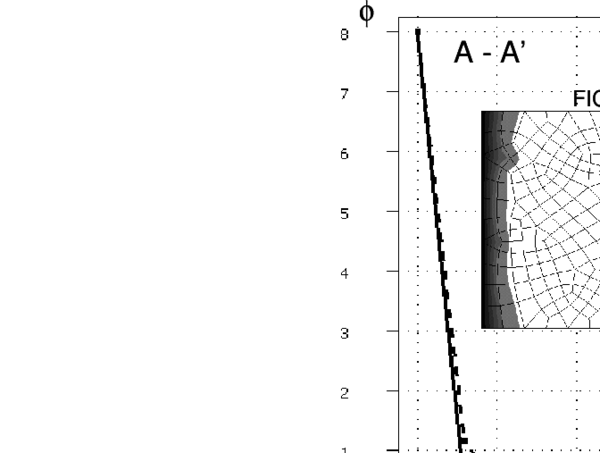Solution of problem of Figure 4 with an unstructured mesh of 209 four node bi-linear quadrilaterals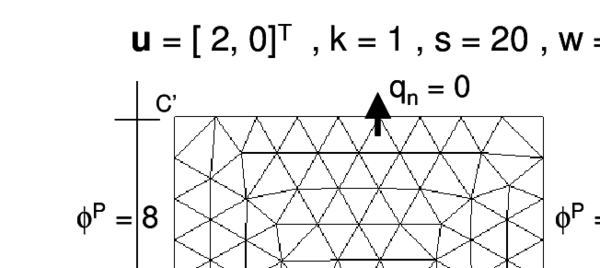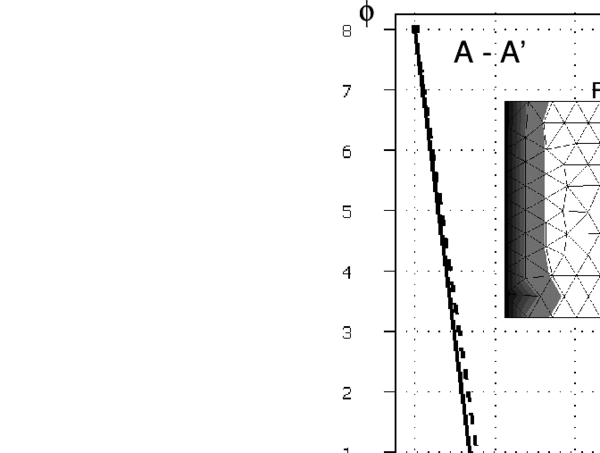Solution of problem of Figure 4 with an unstructured mesh of 176 three node linear triangles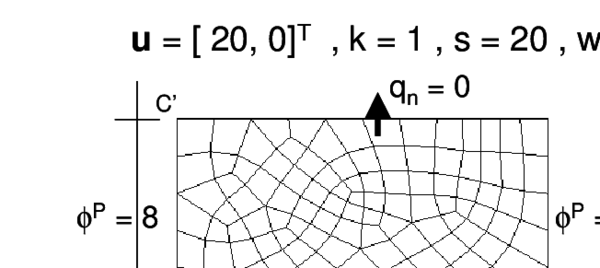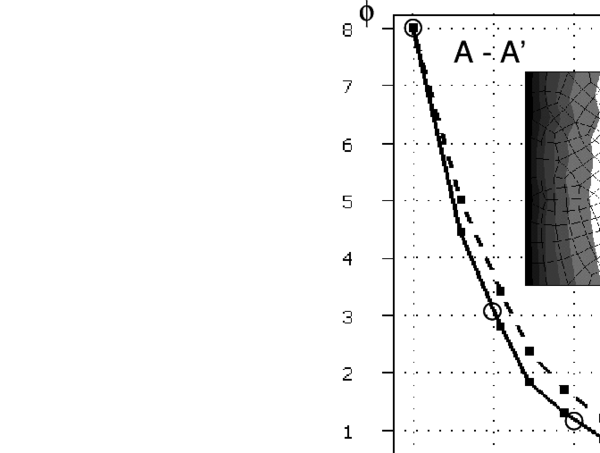Solution of problem of Figure 6 with an unstructured mesh of 209 four node bi-linear quadrilaterals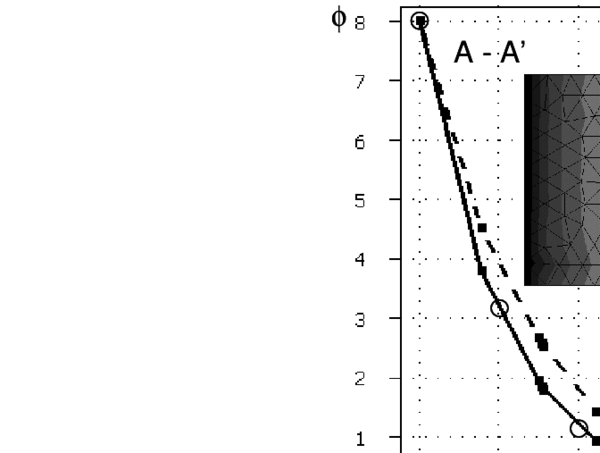Solution of problem of Figure 6 with an unstructured mesh of 176 three node triangles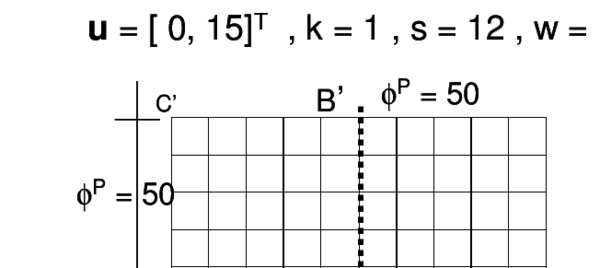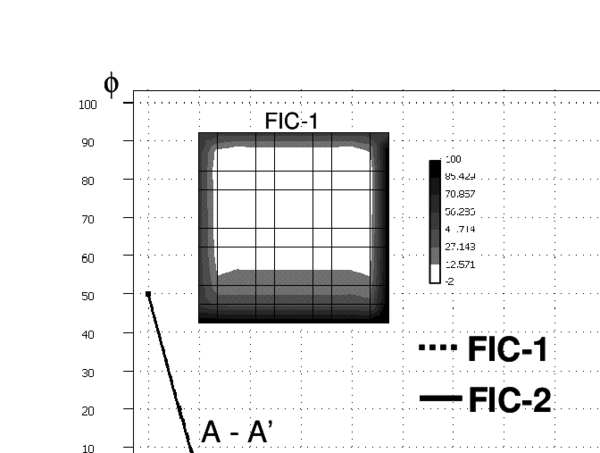2D advection-diffusion-absorption problem over a square domain of size equal to 10 units. $\phi ^{p}=50$ along $x=0$ and $y=10$ and $\phi ^{p}=100$ along $x=10$ and $y=0$, ${u}=[0,15]^{T}$, $k=1$, $s=12$, $w=12$, $\gamma _{x}=0$ and $\gamma _{y}=7.5$. Galerkin and FIC solutions for a mesh of $10\times 10$ four node bi-linear square elements.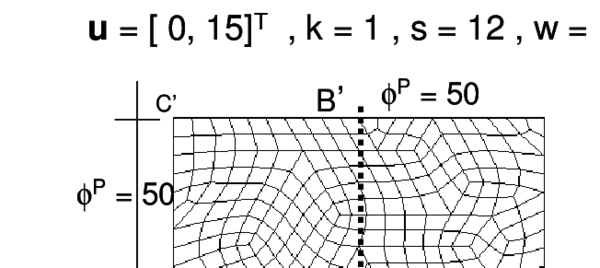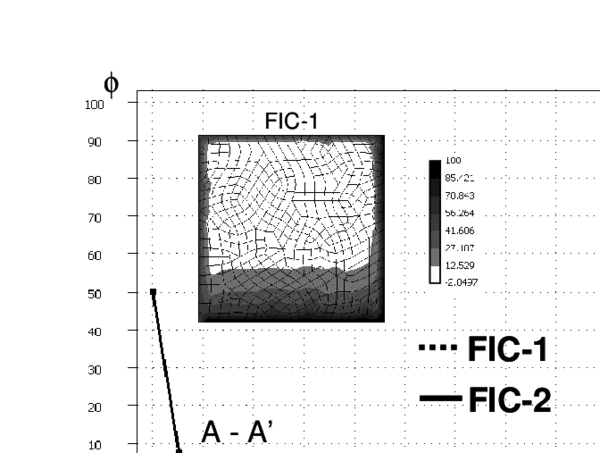Solution of the problem of Figure 12 with an unstructured mesh of 432 four node bi-linear quadrilaterals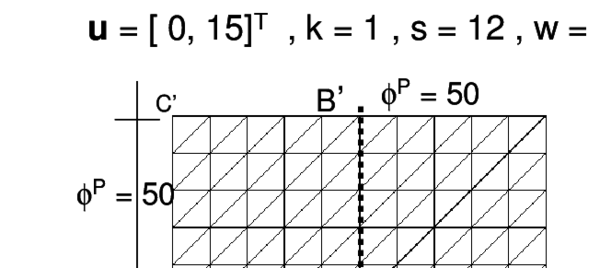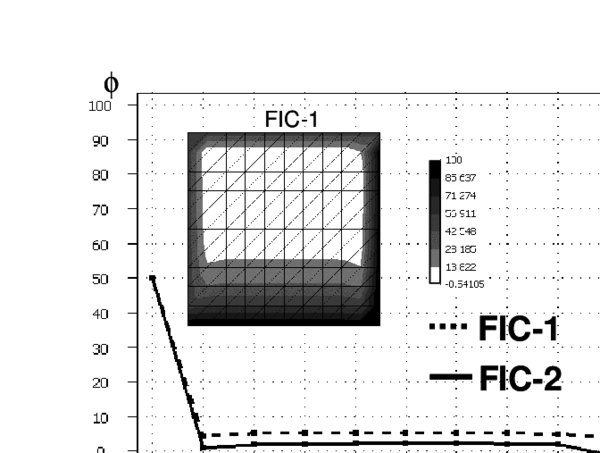Solution of problem of Figure 12 with an structured mesh of $10\times 10\times 2$ three node linear triangles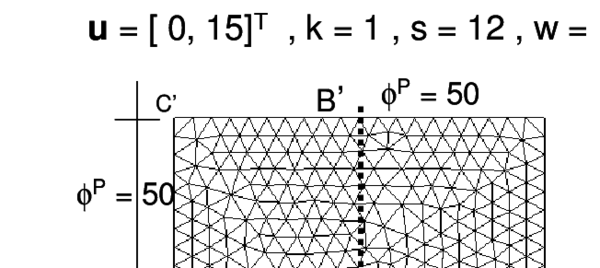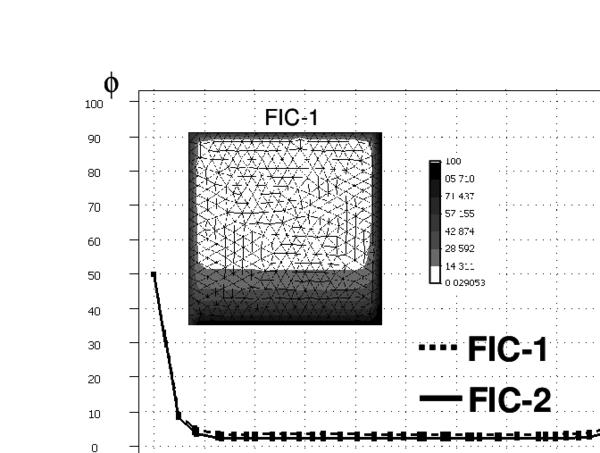Solution of problem of Figure 12 with an unstructured mesh of 780 three node triangles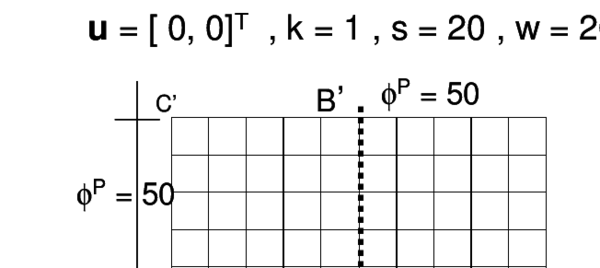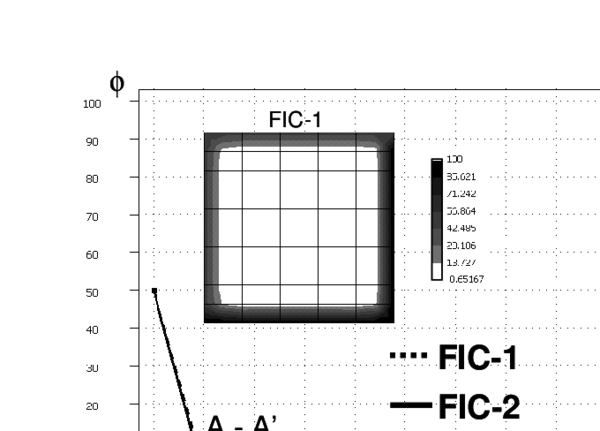Diffusive-absorptive problem over a square domain of size equal to 10 units. $\phi ^{p}=50$ over $x=0$ and $y=10$ and $\phi ^{p}=100$ over $x=10$ and $y=0$, ${u}=[0,0]^{T}$, $k=1$, $s=20$, $w=20$, $\gamma _{x}=0$ and $\gamma _{y}=0$. Galerkin and FIC solutions obtained with structured meshes of four node quadrilaterals and linear triangles.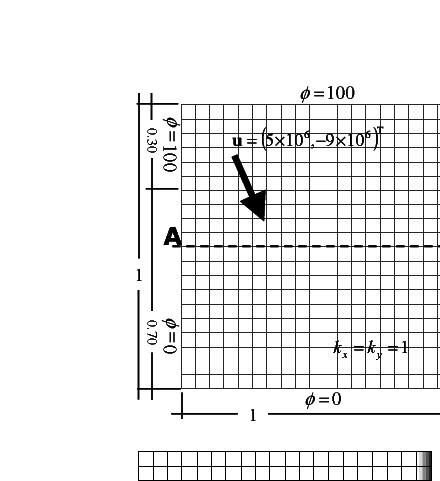Square domain with non uniform Dirichlet conditions, downwards diagonal velocity and zero source. SUPG and FIC solutions obtained with a structured mesh of $20\times 20$ linear four node square elements

## 8 CONCLUSIONS

The FIC-FEM formulation presented allows to obtain a stabilized and accurate solution for the advection-diffusion-absorption equation. For the 1D problem the formulation is equivalent to adding a non-linear diffusion term to the standard discretized equations which is governed by a single stabilization parameter. The use of the constant critical value of the 1D stabilization parameter provides a stabilized numerical solution in a single step. A more accurate (less diffusive) solution can be obtained using the two step iterative scheme proposed.

The equivalence of the FIC method with a nonlinear stabilizing diffusion term extends naturally to multidimensional problems using structured and unstructured meshes. The key step is to express the governing equations of the FIC formulation in the principal curvature directions of the solution. The resulting FIC equation is equivalent to adding a nonlinear diffusion matrix to the infinitessimal governing equations. The solution process becomes non linear and a simple iterative algorithm has been presented. The approximation of the main principal curvature direction by that of the gradient vector simplifies the computations in the iterative scheme. Excellent results have been obtained for all the 2D problems solved in just two iterations for structured and nonstructured meshes.

It is remarkable that, similarly to the 1D case, good stabilized results are obtained in the first iteration of the scheme proposed (FIC-1 results) and this may be sufficient for many practical cases. More accurate (less diffusive) results are obtained by performing a second iteration at a relatively small additional computational cost.

## ACKNOWLEDGEMENTS

The authors also thank Profs. C. Felippa and S.R. Idelsohn for many useful discussions.

This work has been sponsored by the Ministerio de Educación y Ciencia of Spain. Plan Nacional, Project numbers: DPI2001-2193, BIA2003-09078-C02-01, and DPI2004-07410-C03-02.

### Document informationPublished on 01/01/2007

DOI: 10.1016/j.compfluid.2005.07.003

### Document Score0

Times cited: 14
Views 43
Recommendations 0